# Linear Regression, Histograms, Variance and Standard Deviation, R-Squared, Decision Tree Regressor, Polynomial Regression, Multiple Regression¶

In our first tutorial we got some insights about the classification of data, how to use a Decision Tree Classifier in order to predict a new instance's class membership, how accuracy can be measured and what K-Folding is and how it works. If you are unfamiliar with one of these terms I recommend you to have a look at the first tutorial since understanding these concepts will help you to understand this tutorial better.

Today we also have a large variety of exciting topics - so let's get started!

## Prepare data¶

Today we will learn more about housing prices in Boston by applying a lot of different analysis tools and methods to a dataset provided by Skelarn. As you might remember, Skelarn always makes everything much easier and less time-consuming for us. Therefore, we don't have to do anything but using its function load_boston() in order to get our data. Fantastic! By printing the data's key values we can have a look at the information that comes along with the data. We can see that one of the keys is 'DESCR' which - you might have guessed it - stands for description. For a better understanding it is always nice to know what the data we use is about. Therefore, we print the description of the data and its attributes.

In :
from sklearn.datasets import load_boston

#dataset keys
print(boston_dataset.keys())

#dataset description
print(boston_dataset.DESCR)

dict_keys(['data', 'target', 'feature_names', 'DESCR', 'filename'])
.. _boston_dataset:

Boston house prices dataset
---------------------------

**Data Set Characteristics:**

:Number of Instances: 506

:Number of Attributes: 13 numeric/categorical predictive. Median Value (attribute 14) is usually the target.

:Attribute Information (in order):
- CRIM     per capita crime rate by town
- ZN       proportion of residential land zoned for lots over 25,000 sq.ft.
- INDUS    proportion of non-retail business acres per town
- CHAS     Charles River dummy variable (= 1 if tract bounds river; 0 otherwise)
- NOX      nitric oxides concentration (parts per 10 million)
- RM       average number of rooms per dwelling
- AGE      proportion of owner-occupied units built prior to 1940
- DIS      weighted distances to five Boston employment centres
- TAX      full-value property-tax rate per $10,000 - PTRATIO pupil-teacher ratio by town - B 1000(Bk - 0.63)^2 where Bk is the proportion of blacks by town - LSTAT % lower status of the population - MEDV Median value of owner-occupied homes in$1000's

:Missing Attribute Values: None

:Creator: Harrison, D. and Rubinfeld, D.L.

This is a copy of UCI ML housing dataset.
https://archive.ics.uci.edu/ml/machine-learning-databases/housing/

This dataset was taken from the StatLib library which is maintained at Carnegie Mellon University.

The Boston house-price data of Harrison, D. and Rubinfeld, D.L. 'Hedonic
prices and the demand for clean air', J. Environ. Economics & Management,
vol.5, 81-102, 1978.   Used in Belsley, Kuh & Welsch, 'Regression diagnostics
...', Wiley, 1980.   N.B. Various transformations are used in the table on
pages 244-261 of the latter.

The Boston house-price data has been used in many machine learning papers that address regression
problems.

.. topic:: References

- Belsley, Kuh & Welsch, 'Regression diagnostics: Identifying Influential Data and Sources of Collinearity', Wiley, 1980. 244-261.
- Quinlan,R. (1993). Combining Instance-Based and Model-Based Learning. In Proceedings on the Tenth International Conference of Machine Learning, 236-243, University of Massachusetts, Amherst. Morgan Kaufmann.



Now that we know what the data is all about, let's put it into a nice Pandas Dataframe. The boston_dataset.data comes without the target variable: the median value of owner-occupied homes in $1000's. Therefore, we add the target variable to our Dataframe to get a good overview about the entire data set. In : import pandas as pd #get data data = pd.DataFrame(boston_dataset.data, columns=boston_dataset.feature_names) #add target variable to data data['MEDV'] = boston_dataset.target #print 5 first rows of our data data.head()  Out: CRIM ZN INDUS CHAS NOX RM AGE DIS RAD TAX PTRATIO B LSTAT MEDV 0 0.00632 18.0 2.31 0.0 0.538 6.575 65.2 4.0900 1.0 296.0 15.3 396.90 4.98 24.0 1 0.02731 0.0 7.07 0.0 0.469 6.421 78.9 4.9671 2.0 242.0 17.8 396.90 9.14 21.6 2 0.02729 0.0 7.07 0.0 0.469 7.185 61.1 4.9671 2.0 242.0 17.8 392.83 4.03 34.7 3 0.03237 0.0 2.18 0.0 0.458 6.998 45.8 6.0622 3.0 222.0 18.7 394.63 2.94 33.4 4 0.06905 0.0 2.18 0.0 0.458 7.147 54.2 6.0622 3.0 222.0 18.7 396.90 5.33 36.2 ## Linear Regression¶ We saw that our data has a lot of features. Nevertheless, before we use all of them in order to make predictions about housing prices in Boston, we first use a single feature. You might wonder why. Well, the only purpose is to learn about different models: we first want to learn everything about Linear Regression before we dive into the even more exciting world of Multiple Regression. So let's start and choose a single feature! Of course, in order to be able to make predictions we do not simply want to use a random feature - we want to use a feature that is strongly correlated to the housing prices. Therefore, let's find out which feature we could use for our Linear Regression model. In order to do so, we calculate a correlation matrix that - as the name indicates - shows us the correlation of each feature to all the other features. Then we visualize it using a function of the Seaborn library which is great for visualizations. The correlations in the correlation matrix are measured with the Pearson correlation coefficient. What is that? Well, as the name coefficient indicates, it is a coefficient - which means something divided by something else: the denominator is the covariance - the sum of the product of the distance of each x-value to the arithmetic mean of all x-values and the distance of each y-value to the arithmetic mean of all y-values. It is divided by the product of the standard deviations of all x and y-values. It looks like this: $$r = \frac{\sum\limits_{i=1}^n(x_i-\overline{x})(y_i-\overline{y})}{\sqrt{\sum\limits_{i=1}^n(x_i-\overline{x})^2\sum\limits_{i=1}^n(y_i-\overline{y})^2}}=\frac{\tilde{s}_{XY}}{\tilde{s}_X\tilde{s}_Y}$$ This results in values between [-1,1]. If the value is close to -1 there is a negative correlation, if the value is close to zero there is no correlation and if the value is close to 1 it indicates a positive correlation. Now, let's have a look at the correlation matrix: With 0.7 it shows a strong correlation between the number of rooms and the prize of the house per dwelling - something we might have been able to guess but by doing so we would have missed out this beautiful matrix. In : import seaborn as sb import matplotlib.pyplot as plt #building matrix that contains all correlations of all features correlation_matrix = data.corr().round(2) #definiton of figure size for our matrix fig, ax = plt.subplots(figsize=(10,8)) # annot = True to print the values inside the square sb.heatmap(data=correlation_matrix, annot = True)  Out: <matplotlib.axes._subplots.AxesSubplot at 0x11dfe50b8>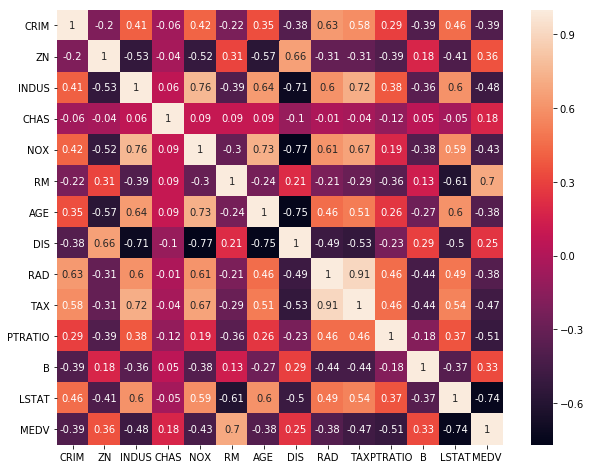Now that we know which feature we want to use for our Linear Regression model, we divide the data into our x-variables and our target variable. In : #Number of Rooms column x_variable = data['RM'] #Median value of owner-occupied homes in$1000's column
y_variable = data['MEDV']


## Histogram¶

Before we start predicting prices, let's have a look at the distribution of our x-variable. We can see that most of the instances have around 6 rooms. Not bad!

In :
#plot histogram
plt.hist(x_variable, color = "pink", rwidth=0.97, bins = 20)

#name for x-axis
plt.xlabel('number of rooms')

#name for y-axis
plt.ylabel('number of instances')

Out:
Text(0, 0.5, 'number of instances')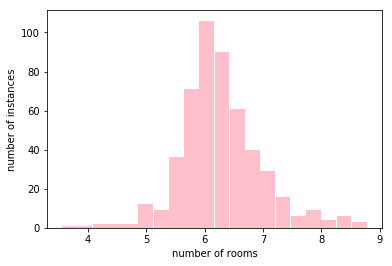Now let's have a look at the distribution of our y-variable: most instances cost around $20000 but there are a few outliners - especially towards the higher prices. In : #plot histogram plt.hist(y_variable, color = "orange", rwidth=0.97, bins = 20) #name for x-axis plt.xlabel('Median value of owner-occupied homes in$1000')

#name for y-axis
plt.ylabel('Number of instances')

Out:
Text(0, 0.5, 'Number of instances')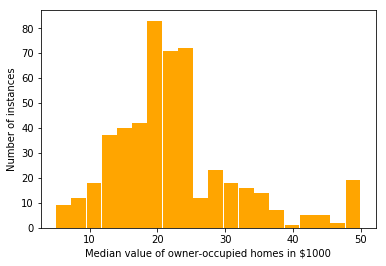## Variance and Standard Deviation¶

The histogram looked nice but we also appreciate precise numbers. Measurements of how strong a variable varies around its arithmetic mean are called Variance and Standard Deviation. Since the Standard Deviation is nothing more but the extracted square root of the Variance, we will first learn how to calculate the Variance. Luckily this is very easy. First, each data point is subtracted from the arithmetic mean of all data points. Then all these distances from the mean are being squared and summed up. This number is then divided by the number of instances. And that's it: $$var(x)=\frac{1}{n}\sum\limits_{i=1}^n(x_i-\overline{x})^2$$ $$var(y)=\frac{1}{n}\sum\limits_{i=1}^n(y_i-\overline{y})^2$$

As already mentioned, by extracting the square root of the variance we get the Standard Deviation. $$\tilde{s}_X=\sqrt{\frac{1}{n}\sum\limits_{i=1}^n(x_i-\overline{x})^2}$$ $$\tilde{s}_Y=\sqrt{\frac{1}{n}\sum\limits_{i=1}^n(y_i-\overline{y})^2}$$ Anyways, as always there are libraries that do all the work for us. So let's look at the results:

In :
import numpy as np

#variance of target variable
variance = np.var(y_variable)
print('Variance: ' +str(round(variance, 2)))

#Standard Deviation of target variable
stand_dev = np.std(y_variable)
print('Standard Deviation: ' + str(round(stand_dev, 2)))

Variance: 84.42
Standard Deviation: 9.19


## Finding the perfect line for a Linear Regression Model¶

Finally we will visualize how the correlation of the number of rooms and the housing prices look like by creating a scatter plot. Looking at the scatter plot we can see that generally spoken with an increase in the number of rooms the price also increases. And voila - there we have our correlation and accordingly our regression!

In :
#definiton of figure size for the plot
fig=plt.figure(figsize=(8, 8))

#display scatter plot
plt.scatter(x_variable, y_variable,  marker='o', s=6, color = "black")

#name y-axis
plt.ylabel('Median value of owner-occupied homes in $1000') #name x-axis plt.xlabel('Number of rooms')  Out: Text(0.5, 0, 'Number of rooms')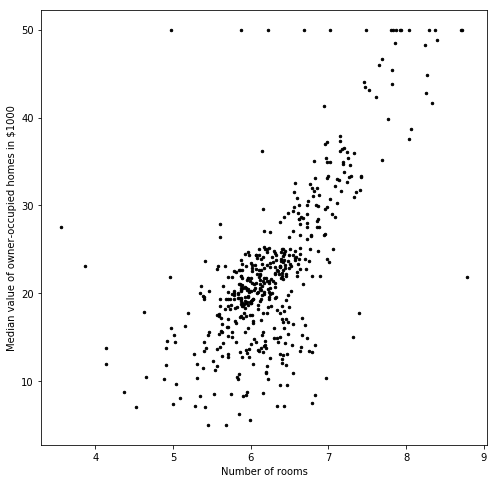Ok, so we have our Regression but where is the "Linear" part of our Linear Regression? Well, we can simply make it linear by - suprise - adding a line to our scatter plot. So let's do it! Well, as usual, before we start, let’s first divide our data into test and training data. In : from sklearn.model_selection import train_test_split #splits into training and test data x_train, x_test, y_train, y_test = train_test_split(x_variable, y_variable, test_size=0.2) # shapes of our data splits print(x_train.shape) print(x_test.shape) print(y_train.shape) print(y_test.shape)  (404,) (102,) (404,) (102,)  Now we use - as always - a library provided by Sklearn in order to create our Linear Regression Model. Then we pass to it our x and y-variables. In order to create the line we let our model predict the y-values which - since our model is a Linear Regression Model - will fall on that line. We will also have a look at the slope and the intercept of that line. In : from sklearn.linear_model import LinearRegression import numpy as np #make a numpy array out of our x_train data to fit the required parameter structure of our Linear Regression Model x_train = np.array(x_train) #make a numpy array out of our y_train data to fit the required parameter structure of our Linear Regression Model y_train = np.array(y_train) #reshape the numpy array of our x_train data to fit the required parameter structure of our Linear Regression Model x_train = x_train.reshape(-1,1) #reshape the numpy array of our x_train data to fit the required parameter structure of our Linear Regression Model y_train = y_train.reshape(-1,1) #create Linear Regression model model = LinearRegression() #fit the model to our data model.fit(x_train, y_train) #get predicted y values which will all fall on a line y_pred= model.predict(x_train) #slope slope = model.coef_ print('Slope: ' + str(slope)) #intercept intercept = model.intercept_ print('Intercept: ' + str(intercept))  Slope: [[9.22694129]] Intercept: [-35.6493485]  Ok, as always Sklearn did a great job and all the work for us and the line really looks as the best line that could ever represent our data. Nevertheless, as always, we are not satisfied by simply looking at results. We also want to know what Sklearn did while finding this perfect line. The idea is to find a line with the least average distance to the data points. Well, in statistics usually there is not the average distance but the average squared distance taken into consideration in order to find that line. These distances are also called squared errors. They are called squared errors because if we use our line to predict the y-value for a certain x-value this error would tell us how far away our prediction probably is from the actual y-value. Therefore, the calculated line is the line with the least amount of squared errors. In : #definiton of figure size for the plot fig=plt.figure(figsize=(8, 8)) #display scatter plot plt.scatter(x_variable, y_variable, marker='o', s=1, color = "blue") #display line plt.plot(x_train, y_pred, color='red') #name x-axis plt.xlabel('Number of rooms') #name y-axis plt.ylabel('Median value of owner-occupied homes in$1000')

#plot title
plt.title('Relationship between RM and prize')

Out:
Text(0.5, 1.0, 'Relationship between RM and prize')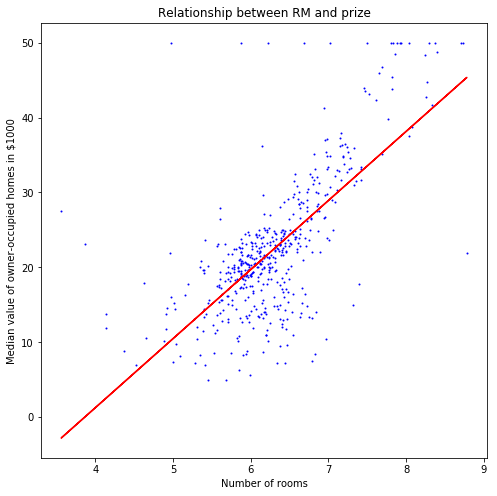Well, this looked nice! Nevertheless, it would also be nice to get some numbers that tell us how well our model actually performs. Therefore, we let our model predict our x_test data.

In :
#make a numpy array out of our x_test data to fit the required parameter structure of our Linear Regression Model
x_test = np.array(x_test)

#make a numpy array out of our y_test data to fit the required parameter structure of our Linear Regression Model
y_test = np.array(y_test)

#reshape the numpy array of our x_test data to fit the required parameter structure of our Linear Regression Model
x_test = x_test.reshape(-1,1)

#reshape the numpy array of our x_test data to fit the required parameter structure of our Linear Regression Model
y_test = y_test.reshape(-1,1)

#get predicted y values which will all fall on a line
y_pred_test_data = model.predict(x_test)


We can now compare the predicted values to the actual values. There are certain similar measurements which will tell us how good the predictions were:

Mean Absolute Error: The average distance of the data points to the line
$$MAE=\frac{1}{n}\sum\limits_{i=1}^n|(y_i-\hat{y_i})|$$ Mean Squared Error: The average squared distance of the data points to the line
$$MSE=\frac{1}{n}\sum\limits_{i=1}^n(y_i-\hat{y_i})^2$$ Root Mean Squared Error: The extracted square root of the Mean Squared Error $$MSE=\sqrt{\frac{1}{n}\sum\limits_{i=1}^n(y_i-\hat{y_i})^2}$$
As always there is a Sklearn library that will provide us with the necessary functions to calculate these values.

In :
from sklearn import metrics

# calculate MAE
mean_abolute_error = metrics.mean_absolute_error(y_test, y_pred_test_data)
print('Mean Absolute Error: ' + str(mean_abolute_error))

#calculate MSE
mean_squared_error = metrics.mean_squared_error(y_test, y_pred_test_data)
print('Mean Squared Error: ' + str(mean_squared_error))

#calculate RMSE
root_mean_squared_error = np.sqrt(metrics.mean_squared_error(y_test, y_pred_test_data))
print('Root Mean Squared Error: ' + str(root_mean_squared_error))

Mean Absolute Error: 4.806676830840376
Mean Squared Error: 58.38915322289628
Root Mean Squared Error: 7.6412795540338845


For a better overview it could also be interesting to compare the predicted price for a house - let's for example say with 6 rooms - to the actual price of a house with the average number of 6 rooms per dwelling. Well, as we could see in our plot that we did earlier, there is not only one house with that amount of rooms. There are actually 312 instances with the average number of 6 rooms per dwelling. As we can see when we calculate the minimum price and the maximum price of the instances at that position, they differ extremely - from 5000 to 50000. No wonder that the calculated MAE, MSE and RMSE were quite high. Nevertheless, when we calculate the average of the prices of the instances at that position, we can see that it is really close to the prediction of our line.

In :
test_rooms = np.array().reshape(1,-1)

five_rooms_pred = model.predict(test_rooms)
print('Model prediction of the price for a house with 6 rooms per dwelling: \n' +str(five_rooms_pred))

prices_six_rooms_real = data.loc[round(data['RM'])== 6, 'MEDV']

num_instances_six_rooms = len(prices_six_rooms_real)
print('Number of instances with the average number of 6 rooms per dwelling:\n' + str(num_instances_six_rooms))

min_price_six_rooms_real = min(data.loc[round(data['RM'])== 6, 'MEDV'])
print('Lowest price of all instances with the average number of 6 rooms per dwelling: \n' + str(min_price_six_rooms_real))

max_price_six_rooms_real = max(data.loc[round(data['RM'])== 6, 'MEDV'])
print('Highest price of all instances with the average number of 6 rooms per dwelling: \n' + str(max_price_six_rooms_real))

mean_num_instances_six_rooms = np.mean(prices_six_rooms_real)
print('Average price of all instances with the average number of 6 rooms per dwelling:\n' + str(mean_num_instances_six_rooms))


Model prediction of the price for a house with 6 rooms per dwelling:
[[19.71229922]]
Number of instances with the average number of 6 rooms per dwelling:
312
Lowest price of all instances with the average number of 6 rooms per dwelling:
5.0
Highest price of all instances with the average number of 6 rooms per dwelling:
50.0
Average price of all instances with the average number of 6 rooms per dwelling:
19.366025641025654


## R-Squared¶

Another important measurement to evaluate a model is R-Squared. R-Squared gives us the percentage of how much of the variation of the target variable can be explained by one or multiple features. In our case this means that R-Squared gives us the percentage of how much of the variation of the housing prices can be explained by the number of rooms. Therefore, we divide the squared distance of the predicted values compared to the actual values by the squared distance of all actual values to their mean and subtract this from 1:
1 - (sum of the squared residuals divided by the variance of the target variable). $$R^2 = 1- \frac{\sum\limits_{i=1}^n(y_i-\hat{y_i})^2}{\sum\limits_{i=1}^n(y_i-\overline{y_i})^2}$$ Of course there is also a library doing this for us. The number below shows us the percentage of the variation in price which can be explained by the number of rooms.

In :
from sklearn.metrics import r2_score

#R-Squared
r_squared = r2_score(y_test, y_pred_test_data)
print(r_squared)

0.3430131062266799


Before we think of a possibility to improve our model, first let's look at the plot with our test data. It does not look very different to the previous one.

In :
#definiton of figure size for the plot
fig=plt.figure(figsize=(8, 8))

#display scatter plot
plt.scatter(x_variable, y_variable, marker='o', s=1, color = "blue")

#sort data ascending
sorted_x_test, sorted_y_test_pred = zip(*sorted(zip(x_test, y_pred_test_data)))

#display line
plt.plot(sorted_x_test, sorted_y_test_pred, color='red')

#name x-axis
plt.xlabel('Number of rooms')

#name y-axis
plt.ylabel('Median value of owner-occupied homes in $1000') #plot title plt.title('Relationship between RM and prize')  Out: Text(0.5, 1.0, 'Relationship between RM and prize')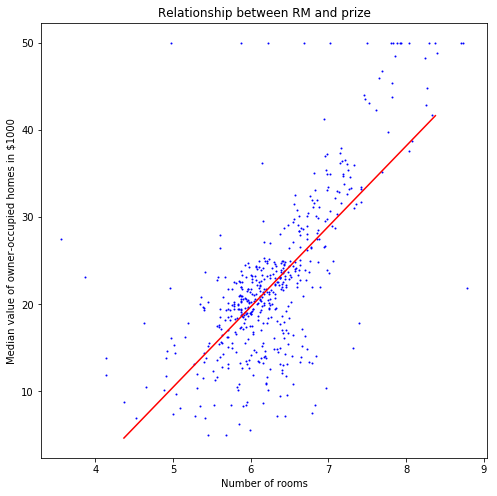We can also visualize the difference between the predicted prices and the acutal prices In : #display data points plt.scatter(y_test, y_pred_test_data, marker='o', s=4) #name x-axis plt.xlabel('actual prices') #name y-axis plt.ylabel('predicted prices') #plot title plt.title('actual versus predicted prices')  Out: Text(0.5, 1.0, 'actual versus predicted prices')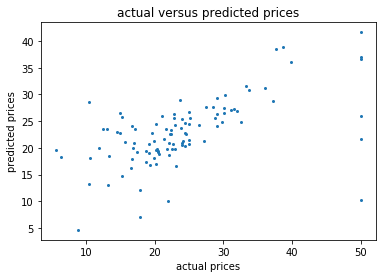## Polynomial Regression¶ Now, let's have a look at whether a line really is the best option to fit our data points - maybe there is actually a better possibility. Could for example a curvy line fit our data better? Luckily we can verify this easily by - yes you got it - using a Sklearn library. By passing the degree-parameter to the constructor we can define which degree our polynomial shall have. While passing the degrees as a parameter it is always important to consider that a high degree can easily lead to overfitting. In this example we use the third degree. Before testing, first let's have a look at how the new line looks like. In : from sklearn.preprocessing import PolynomialFeatures #create PolynomialFeatures object poly = PolynomialFeatures(degree = 3) #prepare x_train data for model x_train_poly = poly.fit_transform(x_train) #prepare y_train data for model y_train_poly = poly.fit_transform(y_pred) #fit model to data model.fit(x_train_poly, y_train) #predicitons y_poly_pred = model.predict(x_train_poly)  In : #definiton of figure size for the plot fig=plt.figure(figsize=(8, 8)) #display scatter plot plt.scatter(x_variable, y_variable, marker='o', s=1, color = "blue") #sort data ascending sorted_x_train, sorted_y_poly_pred = zip(*sorted(zip(x_train, y_poly_pred))) #display line plt.plot(sorted_x_train, sorted_y_poly_pred, color='red') #name x-axis plt.xlabel('Number of rooms') #name y-axis plt.ylabel('Median value of owner-occupied homes in$1000')

# plot title
plt.title('Relationship between RM and prize')

Out:
Text(0.5,1,'Relationship between RM and prize')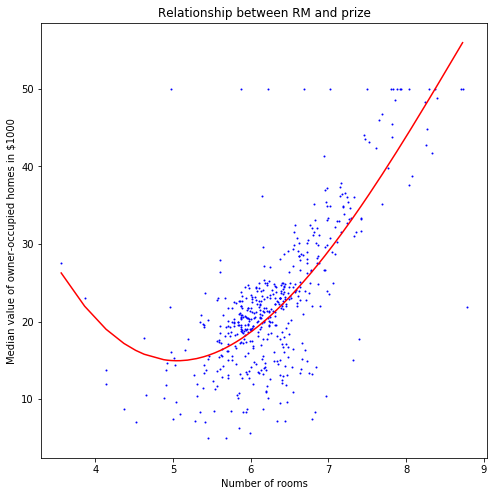Ok, after looking at this beautiful curvy line we can use the same measurements as we did before in order to find out how good our model is. Yay! We can see that the Mean Absolute Error became slightly smaller.

In :
#transform data for predicitons
x_test_poly = poly.fit_transform(x_test)
y_test_poly = poly.fit_transform(y_poly_pred)

#predictions
y_pred_poly_test = model.predict(x_test_poly)

# calculate MAE, MSE, RMSE
mean_abolute_error_poly = metrics.mean_absolute_error(y_test, y_pred_poly_test)
print('Mean Absolute Error Polynomial: ' + str(mean_abolute_error_poly))
mean_squared_error_poly = metrics.mean_squared_error(y_test, y_pred_poly_test)
print('Mean Squared Error Polynomial: ' + str(mean_squared_error_poly))
root_mean_squared_error_poly = np.sqrt(metrics.mean_squared_error(y_test, y_pred_poly_test))
print('Root Mean Squared Error Polynomial: ' + str(root_mean_squared_error_poly))

Mean Absolute Error Polynomial: 4.715846840736583
Mean Squared Error Polynomial: 52.97573762325758
Root Mean Squared Error Polynomial: 7.2784433516554605


## Decision Tree Regressor¶

A non linear method to predict Regression is the Decison Tree Regressor. We already learned about Decision Trees in the preovious tutorial. Nevertheless, the Decison Tree Regressor works slightly different: Instead of using Entropy or Gini Impurity for the splits, the Standard Deviation is taken into consideration in order to do the splits. We could then still improve that model by using GridSearchCV provided by Sklearn as we did in the previous tutorial on Descison Trees in order to find the best parameters for our Descision Tree Regressor model. Nevertheless, as we already know how that works and as there are still other things to learn let's be lazy and skip this for today.

In :
from sklearn.tree import DecisionTreeRegressor
from sklearn.model_selection import cross_val_score

#decision tree regressor object
tree_regressor = DecisionTreeRegressor( max_depth = 2)

# fit the model to the data
tree_regressor.fit(x_train, y_train)

#predicitons
y_tree_pred = tree_regressor.predict(x_test)

#negative absolute errors with 10 cross validations
cross_val_score_desc_tree_regressor = cross_val_score(tree_regressor, boston_dataset.data, boston_dataset.target, cv =10, scoring='neg_mean_absolute_error')
print('negative absolute errors on 10 cross validations: ' + str(cross_val_score_desc_tree_regressor))

#mean negative absolute error
mean_abs_error_desc_tree_regressor = np.mean(cross_val_score_desc_tree_regressor)
print('mean negative absolute error: ' + str(mean_abs_error_desc_tree_regressor))

fig=plt.figure(figsize=(10, 10))

#display data points
plt.scatter(x_train, y_train, edgecolor="black",
c="darkorange", label="data")

#sort data ascending
sorted_x_test, sorted_y_tree_pred = zip(*sorted(zip(x_test,y_tree_pred)))

#display model
plt.plot(sorted_x_test, sorted_y_tree_pred, color="cornflowerblue",
label="max_depth=2", linewidth=2)

#name x-axis
plt.xlabel("Number of rooms")

#name y-axis
plt.ylabel("Median value of owner-occupied homes in \$1000")

#plot title
plt.title("Decision Tree Regression")

#display legend
plt.legend()

negative absolute errors on 10 cross validations: [-2.99422322 -3.64627899 -3.07436455 -5.5645498  -4.58856995 -5.66366577
-5.14234518 -7.55768811 -4.57625943 -3.36966145]
mean negative absolute error: -4.617760645574099

Out:
<matplotlib.legend.Legend at 0x11ead0470>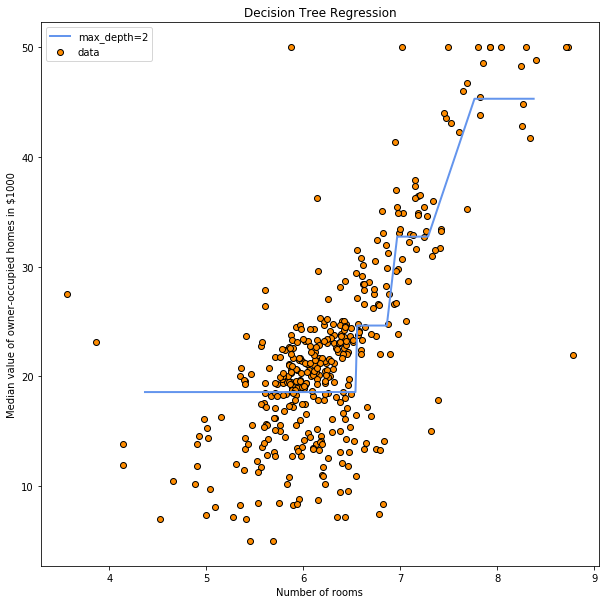As in the previous tutorial we can create an image of our Decision Tree Regressor which can then be found within the folder of this document.

In :
from sklearn.tree import export_graphviz
export_graphviz(tree_regressor, out_file='tree_housing.dot', feature_names=['Number of rooms'])

#Export dot to png
from subprocess import check_call
check_call(['dot','-Tpng','tree_housing.dot','-o','tree_housing.png'])

Out:
0

## Multiple Regression¶

Great! Now we learned a lot about Linear Regression and even learned about a non Linear Model in order to predict Regression. Nevertheless, with Mean Absolute Errors betweeen 4000-5000 dollars we can still not be very satisfied with our predicitons. Why are our predicitions that far away from reality? Well, we actually already know the answer: when we did the test how much a house with 6 rooms costs we had 312 instances with a price range of 5000-50000 dollars. Therefore, apparently, it is not enough to take only the number of rooms into account in order to make a realistic predicition about housing prices. Thus, we will now include the other features which we had left out so far for better predicitions.

In :
#all feature columns
x_variables = data.loc[:, data.columns != 'MEDV']

#target variable
y_variable = data['MEDV']


We can now use the same library as we did before in order to make more accurate predictions - hopefully. Let's first split the data into test and training data.

In :
#training and test data
x_train_multiple, x_test_multiple, y_train_multiple, y_test_multiple = train_test_split(x_variables, y_variable, test_size=0.2)


Let's do a new model and this time fit it to the data that contains all features. How does this work? Well, since we have several features instead of one single feature now, the linear equation that looked like this: $$y = m*x + t$$ became something like this: $$y = m1*x1 +m2*x2 + m3*x3... + t$$ The idea behind finding the perfect coefficients m1,m2,m3... is still the same as before: By using the error function we look for a function with the least amount of squared errors and therefore the least average squared distance to the "line". Due to the many different coefficients and therefore very difficult and time-consuming calculations we are very lucky to live in a world with computers and libraries that are able to do this for us. Since the visualization would now be multidimensional with the amount of dimensions equal to the amount of features, it is impossible to create such a beautiful plot as we did before. Nevertheless, something we can do is to have a look at the calculated coefficients. Generally spoken, the closer the coefficient is to zero, the less important the feature is for our model and our predictions. Therefore, a higher amount of features does usually not degrade the predictions of the model since a small coefficient simply gives less weight to the less important features. Thus, more features usually increase the precision of the model.

In :
#create new LinearRegression object
model_multiple = model = LinearRegression()

#fit model to data
model_multiple.fit(x_train_multiple, y_train_multiple)

#calculated coefficients
coef_multiple = model_multiple.coef_

#DataFrame of features and their coefficients
pd.DataFrame(boston_dataset.feature_names, coef_multiple)

Out:
0
-0.117419 CRIM
0.044571 ZN
-0.003076 INDUS
2.024087 CHAS
-16.125821 NOX
3.727402 RM
-0.000307 AGE
-1.475395 DIS
-0.015624 TAX
-0.930403 PTRATIO
0.006783 B
-0.520741 LSTAT

Let's do some predicitions and find out how well our model works.

In :
#predicitons
y_pred_multiple = model_multiple.predict(x_test_multiple)


As expected, we can see that by adding more features to the model we improved our predicitons.

In :
# calculate MAE, MSE, RMSE
mean_abolute_error_multiple = metrics.mean_absolute_error(y_test_multiple, y_pred_multiple)
print('Mean Absolute Error Multiple Regression: ' + str(mean_abolute_error_multiple))
mean_squared_error_multiple = metrics.mean_squared_error(y_test_multiple, y_pred_multiple)
print('Mean Squared Error Multiple Regression: ' + str(mean_squared_error_multiple))
root_mean_squared_error_multiple = np.sqrt(metrics.mean_squared_error(y_test_multiple, y_pred_multiple))
print('Root Mean Squared Error Multiple Regression: ' + str(root_mean_squared_error_multiple))

Mean Absolute Error Multiple Regression: 3.461371390397248
Mean Squared Error Multiple Regression: 25.36757791852997
Root Mean Squared Error Multiple Regression: 5.036623662586869


We can not plot the multiple regression but we can plot the difference between the predicted and actual prices. Looks much better than when we did the same for the predicitons based on one feature.

In :
#display data points
plt.scatter(y_test_multiple, y_pred_multiple, marker='o', s=4)

#name x-axis
plt.xlabel('real price')

#name y-axis
plt.ylabel('predicted price')

#plot title
plt.title('predicted versus real prices')

Out:
Text(0.5, 1.0, 'predicted versus real prices')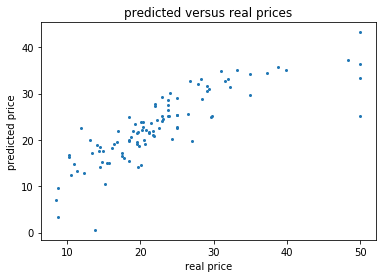That's it for today - but don't be sad! Next time we will learn plenty of other exciting stuff!